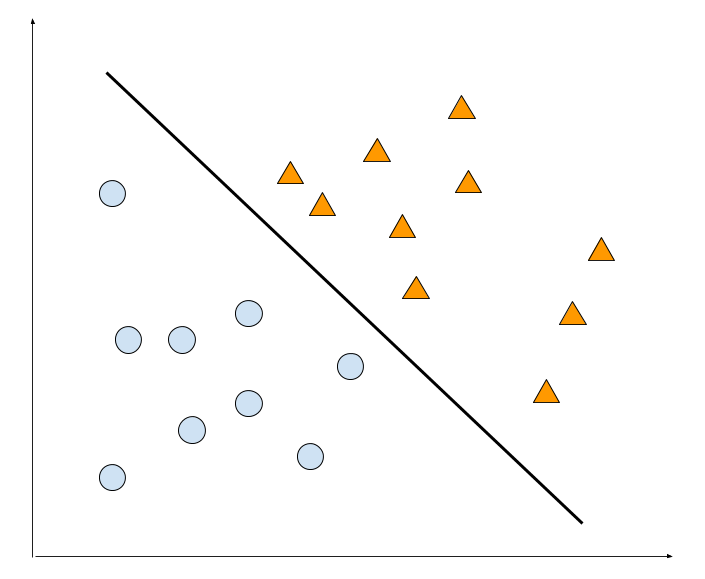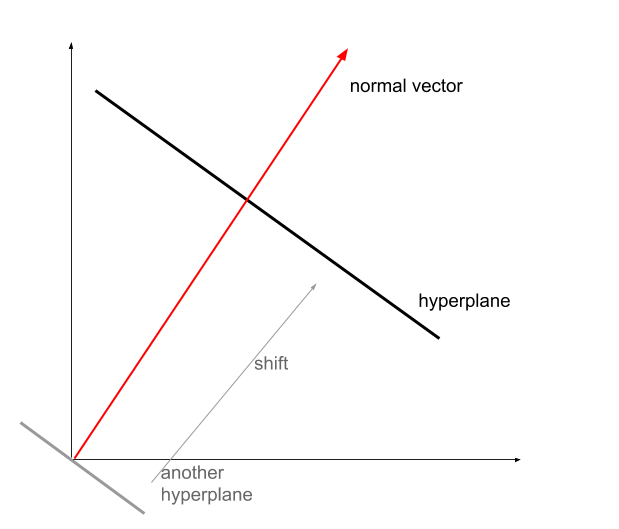SVM is calculating a hyperplane to separate the data points into groups according to the label.

Hyperplane

A hyperplane is defined to be of the following form

$$\begin{equation} \boldsymbol{\beta} \cdot \mathbf x = \beta_0. \end{equation}$$

where $\boldsymbol\beta$ is the normal vector to the plane and is required to be constant.

It is straight forward to show that the distance $d$ from an arbitrary point $\mathbf x’$ to the hyperplane is

$$\begin{equation} d = \boldsymbol\beta \cdot \mathbf x’ - \beta_0. \end{equation}$$

## A Few Key Concepts in SVM

Though the concept of SVM is simple, one might find the algorithm to be quite complicated at first glance.

### Which hyperplane to choose

Suppose we have two classes in our dataset, class A and B, our hyperplane will separate the two classes.

The plane has to make sure that most data points of class A and B are on the two sides of the hyperplane.

1. Lines that are going though at least one point of class A and one point of class B. The examples are shown as dashed and dot-dashed lines.
2. Lines that are going though the edge points of class A. It could be defined later. so we are thinking about lines shown as dotted lines.

Those are absurd limiting choices. However those places us at a position that we might need a plane that has a fare distance between the two classes. Our intuition tells that the hyperplane might not work for those data points close to the hyperplane. That being said, we are more confident if the hyperplane is far away from all data points.

Maybe we could require the hyperplane to be equally far away from the two classes. Here we define the distance between a hyperplane and a group data points to be the smallest distance between the hyperplane and data points. This distance is called the margin.Maybe this one? We calculate the distance between the data points of class A and the hyperplane, and we find the smallest distance two be $d_{A,min}$. Meanwhile we calculate the distance between the hyperplane the the data points of class B, and denote it as $d_{B,min}$. We should find $d_{A, min} = d_{B, min}$. This is the max margin stragety.

We would like to take the extreme limits, again, to understand which hyperplane works the best for the classification problem.

### How is the hyperplane being used

The hyperplane could be represented with a normal vector $\hat{\mathbf n}$ and a shift $\beta_0$.

Why?Fixing a hyperplane: given a normal vector, we could determine the direction of the hyperplane. However, we are also free to move the hyperplane along the normal vector. To fix the hyperplane, we have to specify a shift.

### Outliers?

Is SVM susceptible to outliers?

### Nonlinearity?

Planted: by ;

L Ma (2018). 'Unsupervised Learning: SVM', Datumorphism, 08 April. Available at: https://datumorphism.leima.is/wiki/machine-learning/unsupervised/svm/.